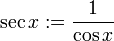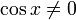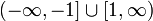# Secant function

This article is about a particular function from a subset of the real numbers to the real numbers. Information about the function, including its domain, range, and key data relating to graphing, differentiation, and integration, is presented in the article.
View a complete list of particular functions on this wiki
For functions involving angles (trigonometric functions, inverse trigonometric functions, etc.) we follow the convention that all angles are measured in radians. Thus, for instance, the angle of$90\,^\circ$ is measured as$\pi/2$.

## Definition

The secant function, denoted$\sec$, is defined as the composite of the reciprocal function and the cosine function.

Explicitly, it is given as follows:$\sec x := \frac{1}{\cos x}$

The domain of the function is the set of all$x \in \R$ for which$\cos x \ne 0$.

## Key data

Item Value
default domain all real numbers except odd integer multiples of$\pi/2$.
range$(-\infty,-1] \cup [1,\infty)$, i.e.,$\{ y \mid |y| \ge 1 \}$
period$2\pi$, i.e.,$360\,^\circ$
vertical asymptotes lines of the form$x = n\pi + \pi/2$.
For$n$ even, left hand limit is$+\infty$ and right hand limit is$-\infty$.
For$n$ odd, left hand limit is$-\infty$ and right hand limit is$+\infty$.
local minimum values and points of attainment local minimum value of 1, attained at integer multiples of$2\pi$.
local maximum values and points of attainment local maximum value of -1, attained at odd integer multiples of$\pi$
important symmetries even function
mirror symmetry about lines of the form$x = n\pi$,$n$ varying over integers.
half turn symmetry about points$(n\pi + \pi/2,0)$,$n$ varying over integers.
first derivative$x \mapsto \sec x \tan x$
second derivative$x \mapsto \sec x \tan^2x + \sec^3x$
first antiderivative$x \mapsto \ln|\sec x + \tan x| + C$.